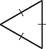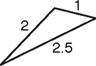Classifying Triangles by Sides

To classify a triangle by its sides means that we look at the side lengths of the triangle and make a determination as to whether it is an: : Equilateral, Isosceles and Scalene. To be an equilateral triangle all three side length must be exactly the same. An Isosceles triangle will have at least 2 side lengths that are the same. If all three sides of the triangle are different then the triangle is scalene.

1. If all the sides are equal (the same length) then the triangle is EQUILATERAL.Example 1: All the sides have a length   Example 2: The "marks" indicate
of 6 mm.that each of the three sides have the same length.

2. If 2 sides of the triangle are the same length then the triangles is an ISOSCELES triangle.Example 1: Two sides have a length of 1   Example 2: The "marks" indicate that 2
and a 3rd side has a different sides have the same length.
length of 1.4.

3. If all three sides of the triangle are a different length then the triangle is a SCALENE triangle.Example 1: All three sides have a     Example 2: If there are no "marks" and no
different length.  numbers indicating length then
all the sides have a different length.

Classifying a triangle is as simple as comparing the sides. If all three sides have the same length then it is an EQUILATERAL triangle, if only two sides have the same length then it is an ISOSCELES triangle and if there are no sides that have the same length then it is a SCALENE triangle. Hint: Remember to look at the "marks" because they represent congruent sides.

 Related Links: Math Geometry Topics Finding the 3rd Angle in a Triangle

To link to this Classifying Triangles by Sides page, copy the following code to your site: